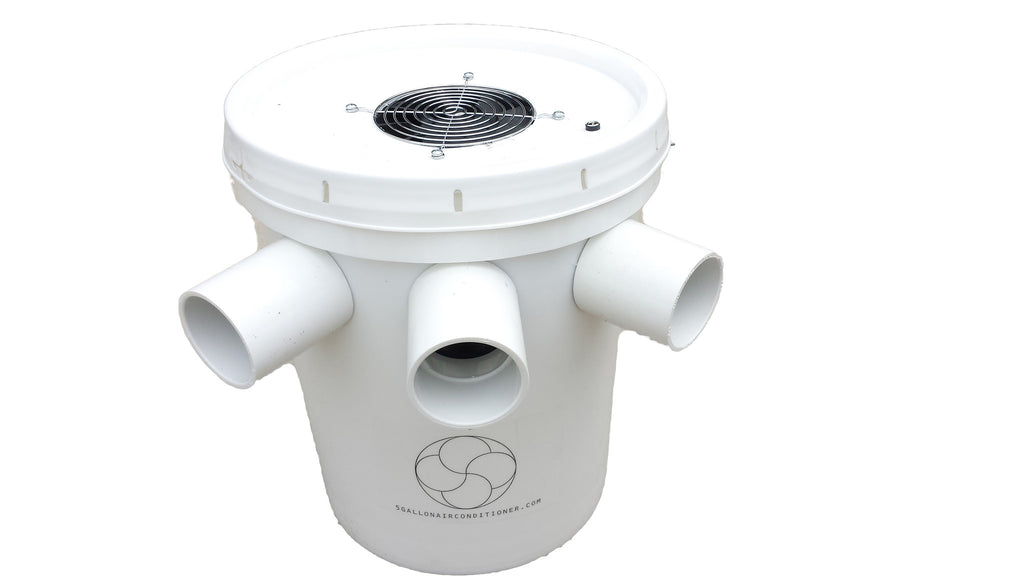# 5 Gallon Air Conditioner - Does it work? What does a TON mean anyway?

Here is the next short blog post in the 5 Gallon Air Conditioner - Does it work series.  The title of this blog post is the "What does a TON mean anyway."

If only there weren't these dang laws of physics to deal with, the world of temperature and thermal comfort would be a lot easier to deal with.

So, we here at 5gallonairconditioner.com get a lot of strange questions and situations presented to us, asking if our 5 Gallon Bucket Air Conditioners will work in X situation.

One of the most common questions is will it work in my car/van/truck/jeep etc, in the deep south with 90% humidity?

In order to answer that question, we have to look at the physics.

In any car/automobile, the air conditioning design engineers have dozens of factors to look at when designing/sizing the AC unit for the car.  Some of those design factors are interior volume, car color, engine heat, exhaust heat, window size, paint adsorption, number of passengers, along with a dozens other factors etc. Because the thermal load in automotive applications is so high, the engineers have to make sure they account for everything "and then some."  For ROUND numbers the AVERAGE automotive AC unit has a rating of 18K BTU/Hr, or what in the industry is 1.5 ton capacity.

So, now we can briefly discuss what TON means in terms of air conditioning.  As has been mentioned in previous blog posts, the latent heat of fusion of ice is 143 BTUs per pound.  So if you have 10 pounds of ice, it would take 1430 BTUs to melt that 10 lbs of ice, and turn it from 31.999 deg F ice to 32.001 deg F water. So, now if you have 1000 lbs of ice, it would take 143,000 BTUs.  If you had 1 TON of ice, it would take 286,000 BTUs.

But, remember that BTUs don't really mean much until you put a time component on them.  So, now if you had 1 TON of ice, it would take 286,000 BTUs to melt it. And if you melted 1 TON of ice in 1 day (24 hrs), then the math works out to 286,000/24 = 11,916 so for round numbers 12,000 BTU/HR = 1 TON.

So, a TON is really a comparison of ice.  Therefore, we can make a direct correlation with our ICE model air conditioners.

So, now an AVERAGE car (we're talking Honda Accord/ Toyota Tacoma/ Ford Fusion etc) has an AC system that is rated at 1.5 Tons. Larger trucks get up to 2 to 2.5 Tons.

So, now with the 5 gallon bucket air conditioner filled with 30 lbs of ice, it is a very easy comparison, 30lbs of ice, is about 1.5% of a ton.  It is 1% of 1.5 Tons.  Remember 1.5 tons = 3000 lbs.

So, the factory air conditioner in an average car, has the cooling capacity of melting 3000 lbs of ice every day. That is the thermal load in an AVERAGE car. So, that calculates out to 125 lbs of ice melted every hour.

So, our little 5 gallon bucket air conditioners filled with 30 lbs of ice are not even in the same league.

So, in that situation, our 5 gallon bucket air conditioners ICE models will not work for long( nor as well as the factory AC unit designed for the car), as our 30 lbs of ice will melt in 1/4 (approximately) of an hour, or for round numbers, every 15 minutes.  With the water coldness and the outside air temperature factored in,  the "ICE model will work" in this environment for approximately 30-40 minutes before the water temperature is too high to have any meaningful difference.

So the answer to the question of, will it work in a car? The answer is YES, but only for about 30-40 minutes.

The next question we get asked a lot is about a small apartment that can't have window units due to fire escape blockage etc, of course in high humidity areas.

Here is the mathematical formula for that calculation.

House(room) square footage multiplied by 25, the product divided by 12,000, and minus 0.5, and that will equal the required tonnage.

So, let's look at a small 1000 sq ft apartment.  Here's how the math works.

1000*25 = 25,000

25,000/12,000= 2.08

2.08-0.5=1.58

So, in this situation, the required size AC unit is 1.5 TONS or 18,000 BTU/hr.  Again, this is the same thermal load as the automotive application above.

In this case, our little 5 gallon bucket air conditioner ICE model will not work.

So, now let's look at a dorm room.  Let's say the dorm room is 200 sq ft.  The math:

200*25 = 5000

5000/12000=0.416

0.416 - 0.5 = negative.

So, in this case, the math works out to where our 5 gallon bucket air conditioner ICE model WILL work.  Now, the key would be to have a constant supply of ice.  But, from a capacity point of view, YES, our 5 gallon bucket air conditioners WILL WORK in this situation.

So, here are again more answers to the question of, 5 gallon bucket air conditioner, does it work?